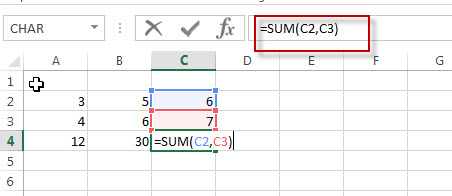# Excel Functions and Formulas Introduction

This post will guide you how to create new function or formulas in excel. How to edit a formula in excel? How to copy or paste a formula in excel 2013?

## What is Excel Formulas?

A formula always starts with an equal sign (=), which can be followed by numbers, math operators (like a + or – sign for addition or subtraction), and built-in Excel functions, which can really expand the power of a formula.

In short, excel formulas is an expression consist of the following elements:  Math operators (+,-,*,/)Cell references (row or column name or range: A2:A5), worksheet functions(such as: date, time, count).

Excel formulas example:

=B1+B2

The above formulas example will add the value of cell B1 to the value of cell B2.

## Create a Formula

If you want to create a formula, you just need to type it in formula bar under excel ribbon.

For example, enter values in A2 and A3 cells, while you clicking A4 cell, you will noticed that the excel formula bar is blank… now enter one formula for the current cell A4, like as: =A2+A3, then the calculated result will be shown in the Cell A4.

1# Input values in A2 and A3 cells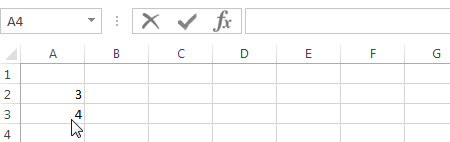2# clicking A4 cell, checking the excel formula bar

3# enter one formula in the formula bar: =A2+A3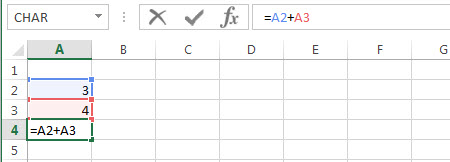4# let’s see the results in the cell A4, it will add the summing value A2 and A3 to A4 cell.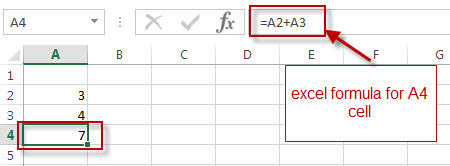## Edit a Formula

If you want to modify excel formula for a special excel cell, you just need to click that cell , then edit its formula in the formula bar. Let’s see the below steps:

1#  click one cell that you want to edit its formula

2# modify the formula as: =A2*A3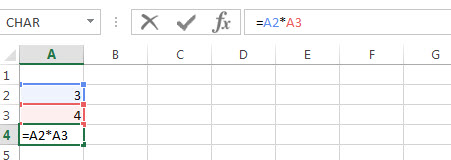3# then press “enter” , let’s see the current result.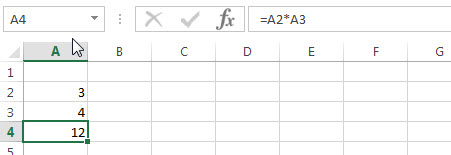## Copy a Formula

If you want to copy one cell formula(A4) to another cell(B4), just following the below steps:

1# clicking the cell A4, right click it and then click “copy” or just press key “CTRL” + “C”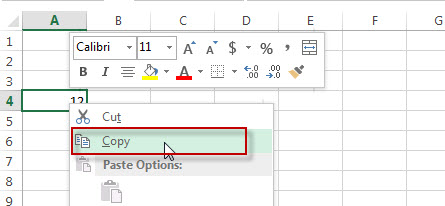2# clicking the cell B4, then right click it , click “Paste Formula” under “Paste Options: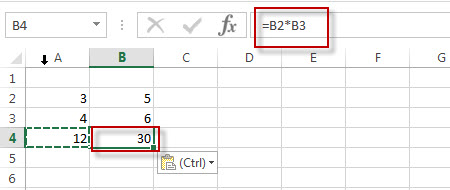3# Let’s see the result: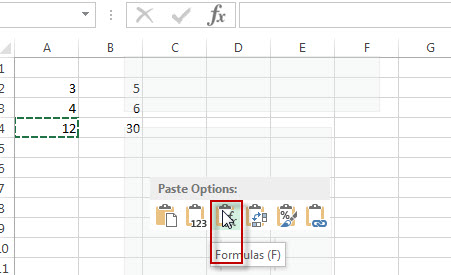## Insert a Function/using Excel built-in function

If you want to insert a function in excel formula bar for one cell, you can just type “=” sign, and then type any alphabet you will see that the searched functions. Or just click “Insert Function” button next to formula bar. Let’s see the below steps:

1# select one cell, such as: C4

2# type “=” sign, then input “S” character, you will see that all functions starting with “S” will show.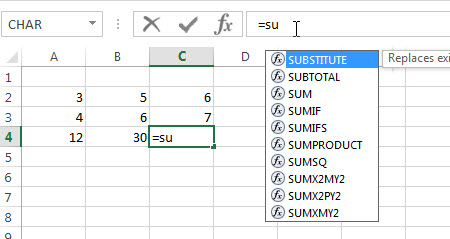Or you can just only click “Insert Function” button to select functions.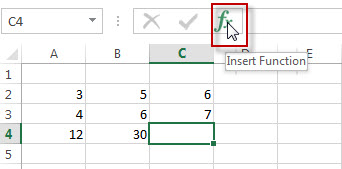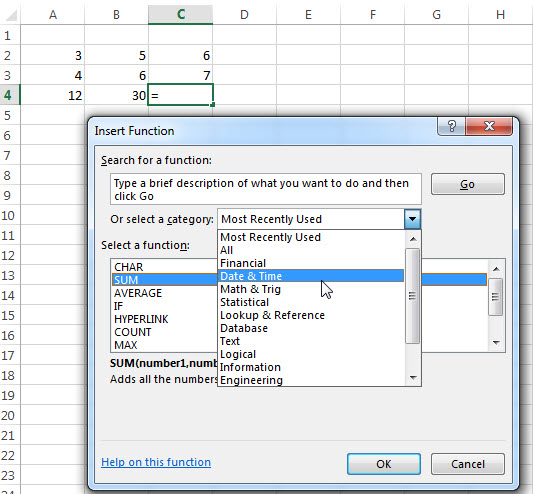Let’s see the results: